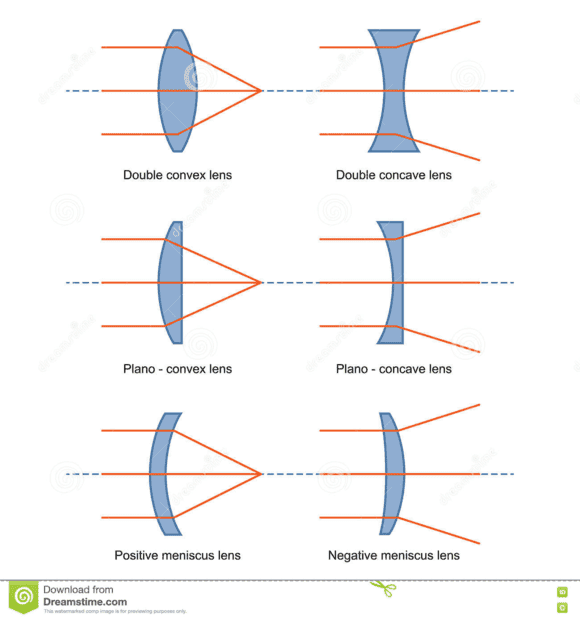# Is gravitational lensing just ordinary refraction?

Paige_Turner
TL;DR Summary
Does 4D spacetime, bent by mass, act like "compressed space" in 3D?
It seems like a strong gravitational field acts like spacetime is denser in some sense. Light passing through a gravitational lens is delayed, just like in a glass lens (which refracts because it's denser than air).

Mentor
While there are similarities between gravitational lensing and refraction by an ordinary glass lens, they are only similarities and can't be taken too far. There is nothing in the actual mathematical model in GR that corresponds to "density of spacetime", so that part of the analogy does not hold.

•vanhees71
Mentor
Summary:: Does 4D spacetime, bent by mass, act like "compressed space" in 3D?

It seems like a strong gravitational field acts like spacetime is denser in some sense. Light passing through a gravitational lens is delayed, just like in a glass lens (which refracts because it's denser than air).
I have never seen any paper that made that analogy.

•vanhees71
Gold Member
Summary:: Does 4D spacetime, bent by mass, act like "compressed space" in 3D?

It seems like a strong gravitational field acts like spacetime is denser in some sense. Light passing through a gravitational lens is delayed, just like in a glass lens (which refracts because it's denser than air).
Light follows similar geometry if you only consider a convex (converging) lens (left-side diagrams).

If this analogy really held, you could theoretically find a galaxy or galaxy cluster that is concave in shape. One would naively expect light passing through volume of space with a concave-shaped mass to diverge (right-side diagrams).•vanhees71